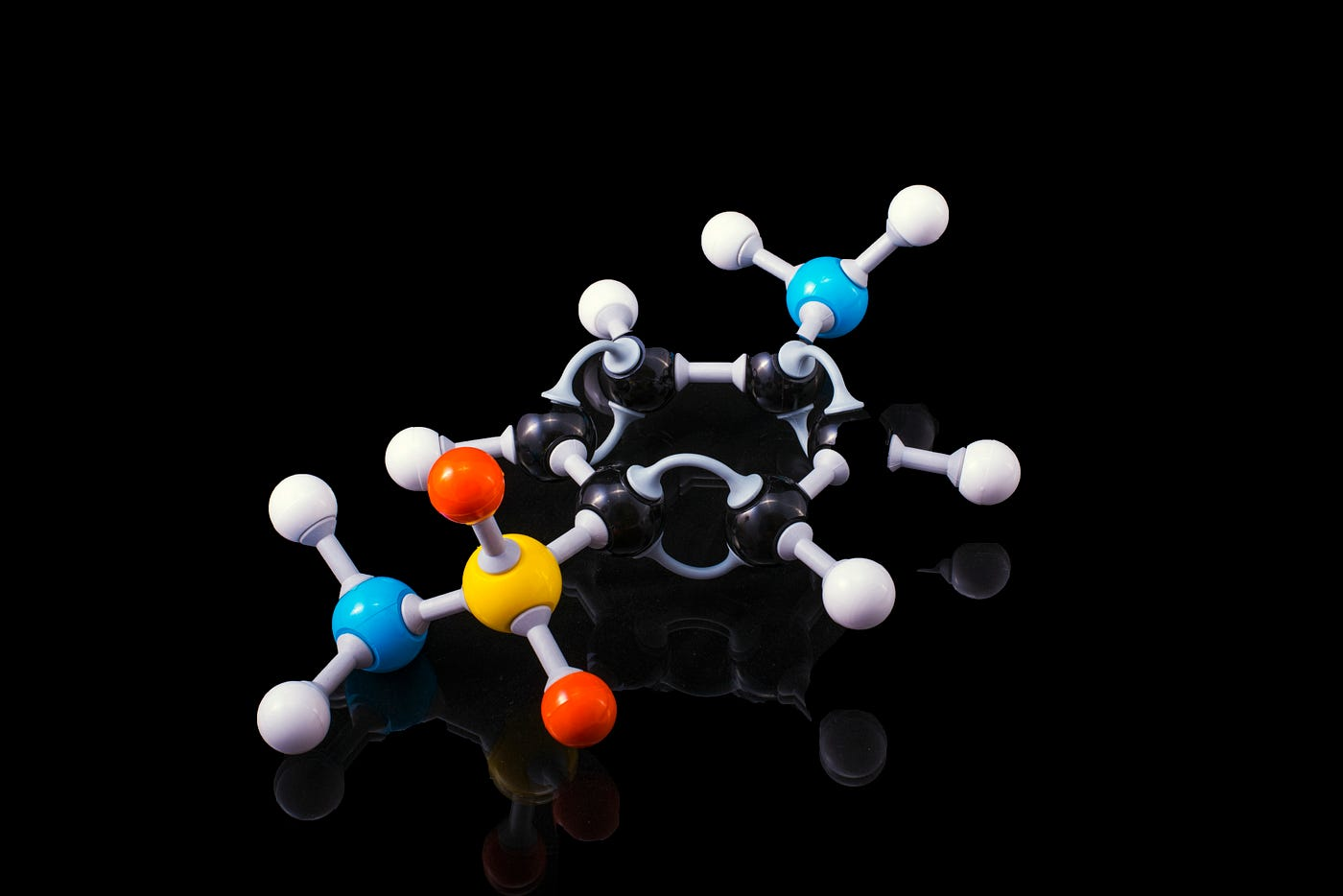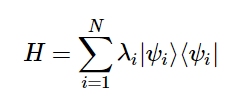# Drug Discovery & Variational Quantum EigensolversPhoto by Terry Vlisidis on Unsplash

It takes at least 10 years and about \$2.6 billion to research and develop a new drug.

Even then, the probability of clinical success is less than 12%.

Over the past year, we’ve seen numerous companies and organizations scramble to develop an effective vaccine. The COVID-19 pandemic highlighted how inefficient our current drug discovery process really is.

Identifying the most optimal drug is the longest and most time-consuming portion of the drug discovery process, simply because of the sheer number of molecular possibilities. An increasing number of electrons results in higher energy electron orbitals, creating a very complicated problem.

Enter quantum computing.

Drugs are composed of tiny molecules, (Which are fundamentally quantum!) which can be simulated on quantum computers, allowing us to build and simulate molecules with an extremely high level of accuracy.

As molecules are essentially quantum systems of their own, their electron configuration states are said to be entangled. Quantum simulations can find the ground state of electron configurations in a molecule, and would consequently give information about its orbitals as well.

# Introduction to VQEs

A hybrid algorithm called the Variational Quantum Eigensolver (VQE) has the ability to find the approximate lowest energy state (AKA ground state) of a molecule very quickly.

Here’s the catch: The VQE algorithm isn’t purely quantum- It’s actually mostly classical. Hybrid algorithms like VQEs use classical computers to do most of the work and rely only on the quantum computer for only a few select tasks.

Although they’re not always going to be 100% accurate, hybrid algorithms like the VQE can give very good approximations, that can be iterated upon.

# How Do VQEs Work?

Here’s some background information:

Finding the ground state energy configuration of a molecule is essentially the same as finding the smallest eigenvalue for a set Hermitian matrix, which is just a matrix that is equivalent to its own conjugate transpose.

The spectral theory states that the eigenvalues of H must be real (Not imaginary), therefore hermitian matrices are perfect for describing the Hamiltonians of quantum systems.

If you need a quick refresher on eigenvectors and eigenvalues, watch this video.

We can describe a Hermitian matrix as:λi is the eigenvalue that corresponds to the eigenvector |ψi⟩

Our expectation value for a quantum state |ψ⟩ would be:

Substituting the first equation into the second gives:

The last equation goes to show that using the eigenvalues of H as weights, you can represent the expectation value of an observable in any state in a linear combination.

Each weight in the equation is equal to or greater than 0, so-

The Variational Method (shown in the equation above) states that the expectation value of any wave function is always greater than or equal to the minimum eigenvalue associated with H.

Therefore, when the Hamiltonian of a system is described by the Hermitian matrix H, the ground state energy configuration of the system is the smallest eigenvalue associated with H.

From here, you would select a wave function (Ansatz), which would be an initial guess for the minimum eigenvalue. Based on the Ansatz, the system calculates an expectation value and iteratively updates it, until it finds the lowest ground state value.

# Using a VQE to find the Ground State Energy and Bond Length of LiH

Using IBM’s SDK Qiskit, I built a VQE to find the bond length associated with the ground state energy of Lithium Hydride.

Watch this video for a detailed walkthrough and explanation of the code, and how it works.

# Let’s Connect!

If you enjoyed reading this article or learned something new, I’d love to connect on LinkedIn. If you’d like to stay updated on my new articles or projects you can subscribe to my monthly newsletter here!

--

--